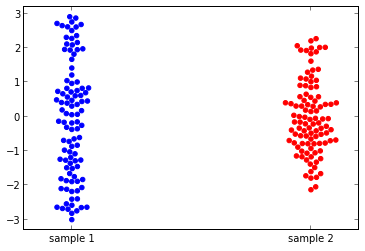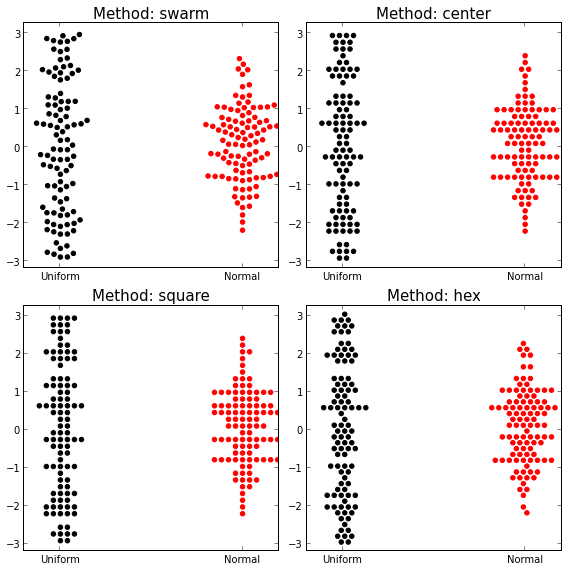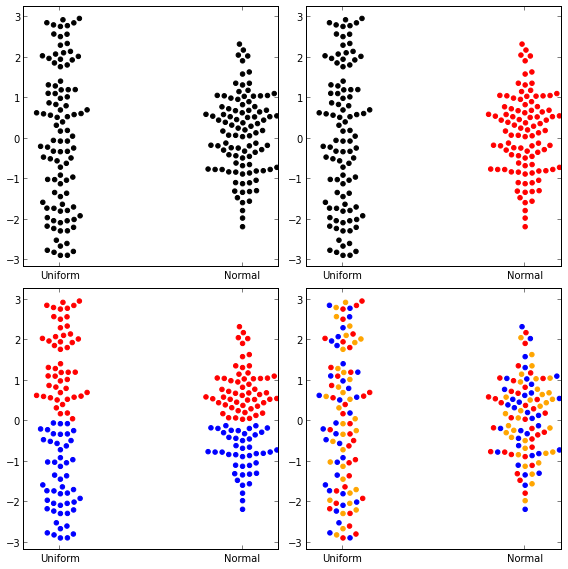## Intro

I have recently migrated all of my plots from R to matplotlib. One cool plot type that I was missing from R was the beeswarm. I tried searching for some Python solutions (some discussion on this can be found here and other places online) but was not satisfied with anything I found (if anyone knows of a good implementation out there, I'd be happy to know about it). I made a simple Python implementation of beeswarm, drawing heavily off of the R beeswarm code. This is available as a python package: https://github.com/mgymrek/pybeeswarm. This was whipped up pretty quickly so it is by no means complete. See wishlist at the bottom.

Note, the code for this IPython notebook can be found here. Also available on nbviewer.

Here's an example before we get started:## Install

To install, simply do:

``` git clone https://github.com/mgymrek/pybeeswarm.git cd pybeeswarm sudo setup.py install```

To test that it worked, open up python and check that `import beeswarm` doesn't give you any errors.

## Basic usage

There is only one function, `beeswarm`:

In :
```from beeswarm import *
help(beeswarm)
```
```Help on function beeswarm in module beeswarm.beeswarm:

beeswarm(values, positions=None, method='swarm', ax=None, s=20, col='black', xlim=None, ylim=None, labels=None)
beeswarm(values, positions=None, method="swarm",
ax=None, s=20, col="black", xlim=None, ylim=None,
labels=None)

Inputs:
* values: an array of a sequence of vectors
* positions: sets the horizontal positions of the swarms.
Ticks and labels are set to match the positions.
If none, set positions to range(len(values))
Default: None
* method: how to jitter the x coordinates. Choose from
"swarm", "hex", "center", "square"
Default: swarm
* ax: use this axis for plotting. If none supplied, make a new one
Default: None
* s: size of points in points^2 (assuming 72 points/inch).
Defautt: 20
* col: color of points. Can be:
- a single string: color all points that color
- a vector of strings length len(values): gives color for each group
- a vector of strings length sum([len(values[i]) for i in range(len(values))])
gives color for each point
- a vector of strings any other length: cycle through the list of colors.
(really pretty if not useful)
Default: "black"
* xlim: tuple giving (xmin, xmax). If not specified, either get
from the supplied ax or recalculate
* ylim: tuple giving (ymin, ymax). If not specified, eiterh get
from the supplied as or recalculate
* labels: list of labels for each group.
Default: range(len(values))

Returns:
* bs: pandas.DataFrame with columns: xorig, yorig, xnew, ynew, color
* ax: the axis used for plotting

```

Here's a small example:

In :
```from beeswarm import *
import matplotlib.pyplot as plt
import numpy as np
d1 = np.random.uniform(low=-3, high=3, size=100)
d2 = np.random.normal(size=100)

bs, ax = beeswarm([d1,d2], method="swarm", labels=["sample 1", "sample 2"], col=["blue","red"])
```## Examples

Here I show what the plots look like for the same examples given on the R beeswarm page for comparison.

### Comparing arrangement methods

In :
```from beeswarm import beeswarm
import matplotlib.pyplot as plt
import numpy as np

d1 = np.random.uniform(low=-3, high=3, size=100)
d2 = np.random.normal(size=100)

fig = plt.figure()
fig.set_size_inches((8,8))
ax1 = plt.subplot(221)
ax2 = plt.subplot(222)
ax3 = plt.subplot(223)
ax4 = plt.subplot(224)
axes = [ax1, ax2, ax3, ax4]
methods = ["swarm","center","square","hex"]
for i in range(len(axes)):
beeswarm([d1, d2], col=["black","red"], method=methods[i], ax=axes[i], labels=["Uniform","Normal"])
axes[i].set_title("Method: %s"%methods[i], size=15)
plt.tight_layout()
```### Specifying colors

You can specify one color for everything, one color per group, one color per point, or cycle through colors, given by the four examples below:

In :
```fig = plt.figure()
fig.set_size_inches((8,8))
ax1 = plt.subplot(221)
ax2 = plt.subplot(222)
ax3 = plt.subplot(223)
ax4 = plt.subplot(224)
beeswarm([d1,d2], method="swarm", labels=["Uniform","Normal"], col="black", ax=ax1)
beeswarm([d1,d2], method="swarm", labels=["Uniform","Normal"], col=["black","red"], ax=ax2)
def GetColor(x):
colors = []
for item in x:
if item > 0: colors.append("red")
else: colors.append("blue")
return colors
colors = GetColor(d1) + GetColor(d2)
beeswarm([d1,d2], method="swarm", labels=["Uniform","Normal"], col=colors, ax=ax3)
beeswarm([d1,d2], method="swarm", labels=["Uniform","Normal"], col=["red","blue","orange"], ax=ax4)
plt.tight_layout()
```## Conclusion

There are several features that the R package has that I didn't implement here:

• "Corral" methods that keep the data from spreading too far horizontally
• The ability to do horizontal vs. vertical plots
• The ability to take in formula objects, just as R methods such as boxplot, lm, etc. take. This can be done in Python using patsy, but I am not very familiar with the package yet so I didn't venture there.

If I come across the need to use these features, (or if anyone would like to add them...) it might happen in the future. For now, I hope those of you missing this package from R will find this useful. Let me know if you have any feedback. Happy plotting!